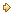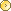Visitors Online: 77 | Monday 27th May 2019

CBSE Guess > Papers > Question Papers > Class XII > 2006 > Economics > Delhi Set - I

Economics — 2006 (Set I — Delhi)

SECTION - A

Q. 1. Answer the following questions: (1 x 4 = 4)

1. When is a good called an ‘inferior good’?
2. Define marginal cost.
3. When is the supply of a commodity called ‘elastic’?
4. Define marginal physical product.

Q. 2. What is meant by consumer’s equilibrium? State its condition in case of a single commodity. (3)

Q. 3. State the ‘total expenditure method’ of measuring price elasticity of demand. (3)

Q. 4. What is meant by returns to a factor ? State the law of diminishing returns to a factor. (3)

Q. 5. State any three causes of a rightward shift of supply curve. (3)

Q. 6. The price elasticity of supply of a commodity is 2. When its price falls from Rs. 10 to Rs. 8 per unit, its quantity supplied falls by 500 units. Calculate the quantity supplied at the reduced price. (4)

Q. 7. What change in total revenue will result in

1. a decrease in marginal revenue, and
2. an increase in marginal revenue? (4)

Q. 8. Explain the problem of ‘what to produce’ with the help of an example.

Or

Why does an economic problem arise? Explain the problem of ‘how to produce’. (4)

Q. 9.Why is the average revenue curve of a firm under perfect competition parallel to x and negatively sloped under monopoly? (4)

Q. 10. The total fixed cost of a firm is Rs. 12. Given below is its marginal cost schedule. Calculate total cost and average variable cost for each given level of output. (6)

 Output (units) 1 2 3 4 5 6 Marginal cost (RS.) 9 7 2 4 8 12

Q. 11. State three causes each for a rightward shift and a leftward shift of demand curve.

Q. 12. How is the equilibrium price and equilibrium quantity of a normal commodity affected by an increase in the income of its buyers? Explain with the help of a diagram.

Or

At a given price of a commodity, there is ‘excess demand’. Is this price an equilibrium price? If not, how will the equilibrium price be reached? (Use diagram) (6)

SECTION - B

Q. 13. Answer the following questions: (1 x 4 = 4)

1. Define macroeconomics.
2. In a government budget, revenue deficit is Rs. 50,000 crores and borrowings are Rs. 75,000 crores. How much is the fiscal deficit’?
3. When will balance of trade show a deficit?
4. Why is the study of the problem of unemployment in India considered a macroeconomic study?

Q. 14. From the following data, calculate “gross value added at factor cost”. (3)

 (Rs. in lakhs) Sales Rent Subsidies Change in stock Purchase of raw materials Profits 180 5 10 15 100 25

Q. 15. Explain the meaning of investment multiplier. What can be its minimum value and why? (3)

Q. 16. Define aggregate demand. State its components. (3)

Q. 17. State the basis of classifying government expenditure into revenue and capital expenditure. Give an example of each. (3)

Q. 18. Explain any one of the following functions of a central bank:

1. Currency authority, and
2. lender of last resort.

Or

Explain the ‘acceptance of deposits’ function of a commercial bank. (4)

Q. 19. Giving reasons, categories the following into revenue receipts and capital receipts: (4)

1. Recovery of loans
2. Corporation tax
3. Dividends on investment made by government
4. Sale of a public sector undertaking

Q. 20. What is meant by foreign exchange rate ? Why does a rise in foreign exchange rate causes a rise in its supply? (4)

Q. 21. Explain the ‘store of value’ function of money. (4)

Q. 22. From the following data calculate national income by

1. income method and
2. expenditure method:(2,4)
 Rs.(in Crores) Private final consumption expenditure Net capital formation Change in stock Compensation of employees Rent Interest Operating surplus Net indirect tax Employers’ contribution to social security schemes Net exports Net factor income from abroad Government final consumption expenditure Consumption of fixed capital 2,000 400 50 1,900 200 150 720 400 100 20 (-) 20 600 100

Or

From the following data calculate: (3,3)

1. Private income, and
2. Personal disposable income
 (Rs. in lakhs) Income from domestic product accruing to the private sector Savings of non-departmental public enterprises Current transfers from government administrative departments Savings of private corporate sector Current transfers from rest of the world Net factor income from abroad Corporation tax Direct personal taxes 4,000 200 150 400 50 (-) 40 60 140

Q. 23. Draw on a diagram a straight line savings curve for an economy. From it derive the consumption curve, explaining the method of derivation. Show a point on the consumption curve at which average-propensity to consume is equal to 1. (6)

Q. 24. Will the following factor incomes be included in domestic factor income of India ? Give reasons for your answer. (6)

1. Compensation of employees to the residents of Japan working in Indian embassy in Japan.
2. Profits earned by a branch of foreign bank in India.
3. Rent received by an Indian resident from Russian embassy in India.
4. Profits earned by a branch of State Bank of India in England.

 Economics 2006 Question Papers Class XII Delhi Outside Delhi Compartment Delhi Compartment Outside DelhiSet ISet ISet ISet I

 CBSE 2006 Question Papers Class XIIEnglishSociologyFunctional EnglishPsychologyMathematicsPhilosopyPhysicsComputer ScienceChemistryEntrepreneurshipBiologyInformatics PracticesGeographyMultimedia & Web TechnologyEconomicsBiotechnologyBusiness StudiesPhysical EducationAccountancyFine ArtsPolitical ScienceHistoryAgriculture